#### STAT 801

Problems: Assignment 3

Suppose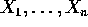are iid real random variables with density f . Let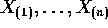be the X 's arranged in increasing order.

1. Find the joint density of.

2. Suppose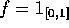. Prove that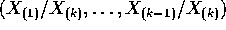is independent of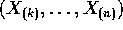.

3. Find the density of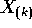.

4. Find the density of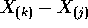.

Suppose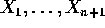are iid exponential. Let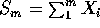.

1. Find the joint density of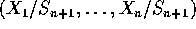.

2. Find the joint density of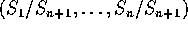.

Supposeare iid N(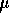,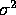). Let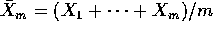. Let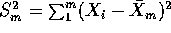.

1. Develop a recurrence relation for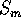and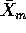, expressingandin terms of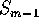and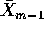.

2. Find the joint density of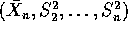.

3. Generate data from N(0,1). By adding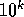to the data for some large values of k compare the numerical performance of these recurrence relations to that of the one pass formula using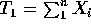,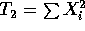and the usual computing formulas for the sample variance.

Suppose X and Y are iid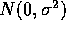.

1. Show that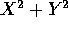and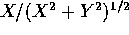are independent.

2. Show that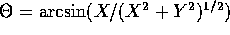is uniformly distributed on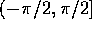.

3. Show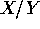is a Cauchy random variable.

Richard Lockhart
Thu Oct 10 22:15:37 PDT 1996